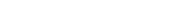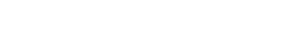Home > Subjects > IT/Technology > Dynamic Programming

# Dynamic Programming

A REPORT SUBMITTED TO THE DEPARTMENT OF SOFTWARE ENGINEERING

##### Acknowledgment

We would like to express our deep gratitude to our research supervisor, for his sublime guidance, enthusiastic encouragement and useful critiques of this presentation. Grateful thanks to him for his advice and assistance in keeping our progress on schedule. We would also like to thank our parents for their support and encouragement throughout our group study.

##### Table of content
• Abstract
• Introduction
1. Dynamic Programming
2. Fibonacci Sequence
3. Chain Matrix Multiplication
4. Knapsack Algorithm
• Literature Review
• Conclusion
• References

#### Abstract

The origin of the term “Dynamic programming” has very little to do with writing code. It was first coined by Richard Bellman in the 1950s, a time when computer programming was an esoteric activity practiced by so few people. Dynamic programming is a very powerful algorithmic paradigm in which a problem is solved by identifying a collection of sub problems and tackling them one by one, smallest first, using the answers to small problems to help figure out larger ones, until the whole lot of them is solved. In the 12th century, a man named Leonardo Fibonacci questioned what the population growth of rabbits would be like under ideal circumstances, such as no predators to eat them, or lack of food and water that would affect the growth rate. The results of this experiment are now known as The Fibonacci Sequence of Numbers or Fibonacci Numbers, and it goes like this, Starting with 1, each new number in the series is simply the sum of the two before it. In dynamic programming we use the principle of optimally suppose we are given a sequence (chain) A1, A2… A of n matrices, and we wish to find the product. The way we parenthesize a chain of matrices can have a dramatic impact on the cost of evaluating the product. This problem is to determine the best way to parenthesize the matrices to minimize the number of multiplications. We are given some items; pack the knapsack to get the maximum total value. Each item has some weight and some value. Total weight that we can carry is no more than some fixed number “W”. So we must consider weights of items as well as their values.

### Introduction Dynamic Programming:

Bellman pioneered the systematic study of dynamic programming in 1950s.The fundamental idea behind dynamic programming is to solve a given problem by solving a series of subproblems. The series of subproblems is conceived in a manner that permits a subsequent subproblem to be solved by combining the solutions of one or more of the subproblems already solved – making dynamic programming a bottom-up approach. The intermediate solutions are held in an array to eliminate repeating work. For optimization problems a dynamic programming algorithm is typically developed in three steps. The first step establishes a recursive formulation that yields the optimal solution to an instance of the problem; the next step computes the value of an optimal solution in a bottom-up fashion; and, the final step constructs an optimal solution in a bottom-up fashion. 

The dynamic programming relies on a “principle of optimality”. This principle states that in an optimal sequence of decisions or choices, each subsequence must also be optimal. For example, in matrix chain multiplication problem, not only the value we are interested in is optimal but all the other entries in the table are also representing optimal. The principle can be related as follows: the optimal solution to a problem is a combination of optimal solutions to some of its subproblems.

#### Fibonacci Sequence:

By definition, the first two numbers in the Fibonacci sequence are 1 and 1, or 0 and 1,depending on the chosen starting point of the sequence, and each subsequent number is the sum of the previous two numbers.

The Fibonacci sequence is the series of numbers:

0, 1, 1, 2, 3, 5, 8, 13, 21, 34…

A general equation we can write for the Fibonacci sequence is:If you take any two successive (one after the other) Fibonacci Numbers, their ratio is very close to the Golden Ratio “φ” which is approximately 1.618034… 

Fibonacci Sequence Applications:

Fibonacci numbers have geometric applications in nature .The most prominent of these is the Fibonacci spiral. Many plants show the Fibonacci numbers in the arrangement of the leaves around the stem. Some pine cones and fir cones also show the numbers, as do daisies and sunflowers.

#### Chain Matrix Multiplication:

If we are given some matrices to multiply, then determine the best order to multiply them so minimize the number of single element multiplications i.e. determine the way the matrices are parenthesized. If we are given a sequence of j matrices, A1, A2, . . . ,Aj. We want to compute the product, A1A2…Aj as quickly as possible. In particular, we want to fully parenthesize the expression above so there are no ambiguities about how the matrices are multiplied. There are many ways to parenthesize the matrices. Each way gives the same output (because of associativity of matrix multiplications).An optimal parenthesization of the product A1A2…Aj splits the product between Ak and Ak+1 for some integer k where 1 ≤ k < j .First compute matrices A1..k and Ak+1..j ; then multiply them to get the final matrix A1..j

• Then total cost to compute Ai..j is:
• Cost to compute A i..k +
• Cost to compute Ak+1..j +
• Cost to multiply Ai..k and Ak+1..j

If “m” is minimum scalar multiplication then general formula is:By using this we can find an optimal parenthesization for j number of matrices (which are to be multiplied.)

Chain Matrix Multiplication Example:

Consider the sequence of three matrices, A1,A2,A3,A4 whose dimensions are given by the sequence 3, 1, 2, 1, 2 (i.e. p0 = 3, p1 = 1, p2 = 2, p3 = 1, p4 = 2) now we want to parenthesize these matrices in order to perform minimum number of multiplications.

In this regrad first we will plot 2 table, first table is ”m” table where m is minimum number of multiplications, second one is ”s” table where ”s” is solution. Number of coloums and rows in both of the table are equal to the number of matrices available in question.Then we will the apply general formula on matrics sequentally. In formula first we will put the value of ”k” equal to ”i” and then we will gradually increase the value till its equal to one less than ”j”.

”k” should not be equal to ”j” because in this case we are going to consider the whole series. If we are going to multiply A1 and A2 then we can put the value of k just equal to 1 which is eqal to ”i”.Hence we will apply the m formula just for once. In the case of multiplication of matrices from A1 to onward A3 then there are 2 values of ”k” i.e. 1 and 2. so we apply the ”m” formula for two times and minimum value will be selected to put in the table and regarding value of ”k” into the ”s” table. Atlast by carefully observing the ”s” table we will parenthesize the given series of matrices. Hence we will solve the above given examle as follows:

m (1, 4) = min((m(1, 1)+m(2, 4)+p0p1p4),

m(1, 2)+m(3, 4)+p0p2p4),

m(1, 3)+m(4, 4)+p0p3p4))

= min (0+4+6,

6+4+12,

5+0+6)

= 10

This minimum is achieved when k = 1

s Table

 1 2 3 4 1 1 1 1 2 2 3 3 3 4

m Table

 1 2 3 4 1 0 6 5 10 2 0 2 4 3 0 4 4 0

Hence an optimal parenthesization is (A1((A2A3)A4))

Knapsack Algorithm:

We are given a set of items, each with a mass and a value, then determine the number of each item to include in a collection so that the total weight is less than or equal to a given limit and the total value is as large as possible. It derives its name from the problem faced by someone who is constrained by a fixed-size knapsack and must fill it with the most valuable items.

#### Knapsack Algorithm Applications:

One early application of knapsack algorithms was in the construction and scoring of tests, in which the test-takers have a choice as to which questions they answer. For small examples it is a fairly simple process to provide the test-takers with such a choice. For example, if an exam contains 12 questions each worth 10 points, the test-taker need only answer 10 questions to achieve a maximum possible score of 100 points. However, on tests with a heterogeneous distribution of point values—i.e. different questions are worth different point values— it is more difficult to provide choices. Feuerman and Weiss proposed a system in which students are given a heterogeneous test with a total of 125 possible points. The students are asked to answer all of the questions to the best of their abilities. Of the possible subsets of problems whose total point values add up to 100, a knapsack algorithm would determine which subset gives each student the highest possible score.

### Dynamic Programming Literature Review

Dynamic programming originated with the work of Bellman and has been applied to problems in operations research, economics, control theory, computer science, and several other areas. The theory has evolved in several directions: discrete vs. continuous states, finite vs. infinite (countable and uncountable) number of states, and deterministic vs. stochastic systems. Not surprisingly, the literature on dynamic programming is enormous.DP is applicable primarily to the types of problems that can be expressed as a sequence of decisions to be made at each of several stages. For other types of problems there are corresponding problem solving methods. These methods include divide – and – conquer, linear and integer programming, and branch – and – bound. Bellman’s Principle of Optimality is an important and Powerful principle for dynamic programming. It is, however, lacking in at least two ways.

(1) It is not stated very rigorously.

(2) The problem of obtaining the functional equations is not even addressed.

To obtain the functional equations, we must rely on methods beyond the scope of the Principle of Optimality. By the mid 1960’s several people had solved the first problem by showing that the Principle of Optimality derives from a monotonicity property of the cost functions. Karp and Held subsequently made considerable progress on the second problem. In their formulation, a combinatorial decision problem is assumed to be expressed in the form of a discrete decision process (DDP). This form is usually a fairly natural way to express the problem. The dynamic programming algorithm to solve the problem, on the other hand, corresponds to a sequential decision process (SDP). Karp and Held describe the transform (if it exists) from a discrete decision process to a sequential decision process.

#### Conclusion

Hence Dynamic programming is a technique for efficiently computing recurrences by storing partial results. Once you understand dynamic programming, it is usually easier to reinvent certain algorithms than try to look them up!

Dynamic programming is one of the most useful algorithmic techniques in practice:

• Morphing in computer graphics.
• Data compression for high density bar codes.
• Designing genes to avoid or contain specified patterns.
##### References:
• https://functionspace.org/articles/32
• https://www.personal.kent.edu/~rmuhamma/Algorithms/MyAlgorithms/Dynamic/dynamicIntro.htm
• jhave.org/learner/misc/fibonacci/fibonacci.shtml
• www.ccs.neu.edu/home/lieber/courses/csg110/sp08/…/dyn-prog.htm‎
• www.cse.ohio-state.edu/~crawfis/cse680/…/CSE680-10DynamicProg.ppt
• cstheory.stackexchange.com/…/if-you-could-rename-dynamic-program.
• www.goldennumber.net/fibonacci-series
• Repository.cmu.edu
• https://www.world-mysteries.com/sci_17.htm
• www.world-mysteries.com